/

### Enterprise Risk Management Formula Book Appendix A.3: Probability Distributions: Distributional mixtures

A random variable is distributed according to a normal mixture distribution if it is of the form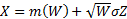where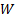and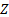are independent random variables,is a non-negative random variable,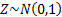and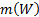is some function of. For example, the t distribution has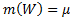and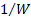being chi-squared with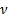degrees of freedom and the standard non-central t distribution has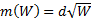where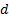is the non-centrality parameter andis chi-squared withdegrees of freedom.

A distributional mixture of normal distributions is to be interpreted more generally as any distribution in which the overall random variable is selected with probability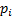from a (typically finite) number of normal random distributions, the’th one of which is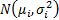for arbitrary constant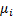and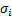. Any univariate distribution can be approximated arbitrarily accurately with a large enough number of underlying normal random distributions. It is contrasted with a linear combination mixture of normal distributions in which the overall random variable is derived by adding together a linear combination of underlying normal random variables, i.e.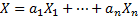.